# LMI Screen Test #5 : 20th - 23rd Nov

## General Screen Test Rules

• Each puzzle will have a time limit, and it has to be solved within the specified time limit.
• There will not be any Puzzle Booklet to download. All the puzzles are to be solved on screen.
• Participants are allowed to draw images / grids on paper and answer on the screen. There is no provision to draw / type / take notes on screen.
• Each puzzle will have certain number of answer options. Participants have to select the answer option using ‘mouse’. Typing is not allowed / needed in ST5.
• There will be 20 puzzles, and they are to be solved within 45 minutes.
• First 10 puzzles will have a time limit of 100 seconds each. Last 10 puzzles will have a time limit of 150 seconds each.

The instructions for example puzzles and test puzzles are absolutely same. If you have read the instructions while viewing examples, you don’t need to read them again while taking the test.
• All puzzles, including the Instructionless, in this Screen Test is based on a theme i.e. maximum. The answer option will always correspond to maximum of "something". We have underlined that "something" in each puzzle.
• Some puzzles may have part of instructions in small font. The text in small font is for completeness of instructions, and should be known to most solvers.

## Puzzles types and examples

### Base points - 25

Which grid has maximum number of shaded cells?The correct answer for the example is C

Which grid has maximum perimeter?The correct answer for the example is B

Which letter is attacked by maximum number of knights?

A knight moves 2-steps in one direction, and 1-step side-way, always moving orthogonally. A knight may jump over another knight.The correct answer for the example is A

Which figure has maximum number of triangles?The correct answer for the example is A

Which equation yields maximum output?

Only addition and subtractions are used.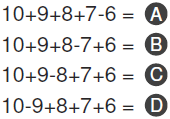The correct answer for the example is A

Which figure has maximum number of circles?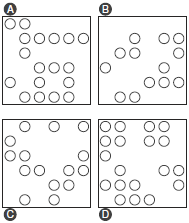The correct answer for the example is D

From which direction, among the marked ones by letters, do you see the maximum number of skyscrapers?

Taller skyscrapers hide smaller ones.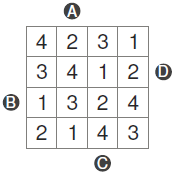The correct answer for the example is B

Which light ray takes maximum number of turns before exiting the grid?

The grid has 2-sided diagonal mirrors. Upon hitting a mirror from either side, the ray makes 90 degrees turn and keeps moving until it hits another mirror or exits out of the grid.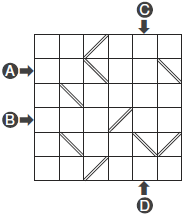The correct answer for the example is C

Shade exactly one cell in each row and each column. What is the maximum possible sum of numbers in the shaded cells?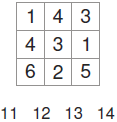The correct answer for the example is 13

All images are rotated clock wise. Which image is rotated the maximum?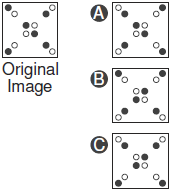The correct answer for the example is C

### Base points - 25

Which letter has maximum weight in the standard Weights puzzle?

Assign digits from the given range to each weight. The horizontal rod indicates that there is equal torque on both sides. The torque of each weight is its weight times its distance from fulcrum. Assume that the strings and rods have negligible weight.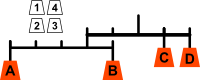The correct answer for the example is C

Starting at the top and moving to adjacent numbers on the row below, create a path to the bottom of the pyramid. What is the maximum possible sum of numbers along the path?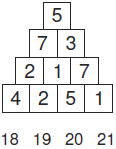The correct answer for the example is 20

Which letter belongs to maximum number of squares?The correct answer for the example is C

What is the maximum number of mines that can be put in the grid, following standard minesweeper rules?

In Minesweeper puzzle, each digit inside the grid represents the number of mines around the 8 cells. Mines are not allowed in cells with digits.The correct answer for the example is 5

Which of the following standard Sudoku grid has maximum solutions?

In a Sudoku grid, 1 through 6 (1 through 4 in the example) should appear exactly once in each row, in each column, in each outlined region.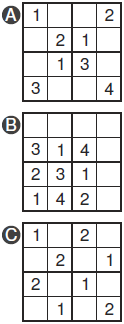The correct answer for the example is C

Which shape appears maximum number of times? Shapes can be rotated / reflected. Cells can be used multiple times.

Black cells can not used while placing the shapes.The correct answer for the example is S

Which Tile appears the maximum number of times? Tiles cannot be rotated or reflected.The correct answer for the example is D

Which letter is pointed by maximum number of arrows?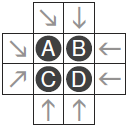The correct answer for the example is B

Which letter is at maximum distance from the marked circle?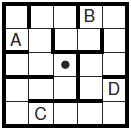The correct answer for the example is D

Two examples will be given. Based on the examples, you need to determine the rules of the puzzle and choose the answer.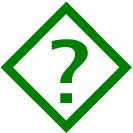## How does the Screen Test interface work?

The easiest way to understand "how the Screen Test works" is to "do the Demo". Here is the link. If you have any questions after doing the demo, please ask in forum.

Here is the summary of how it works, for those who are not doing the demo. There are 3 modes in the Screen Test

• Viewing the examples - You just view the rules and examples
• Taking the actual Test
• Reviewing your submissions - only after you take the test
To take the actual test,